Publicité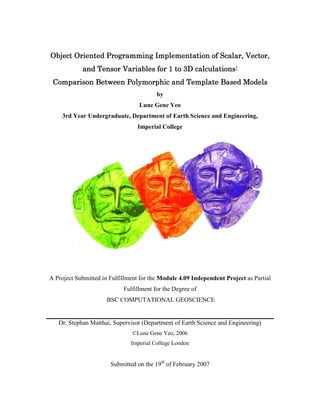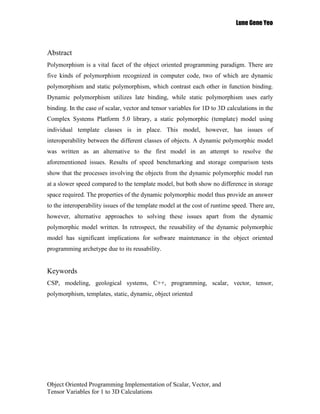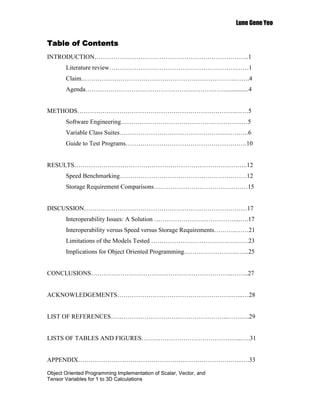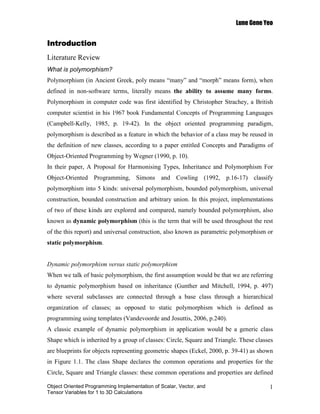Publicité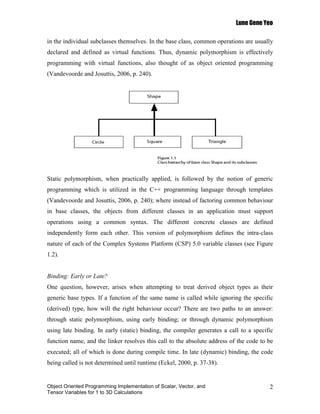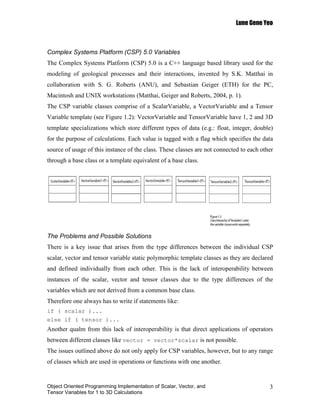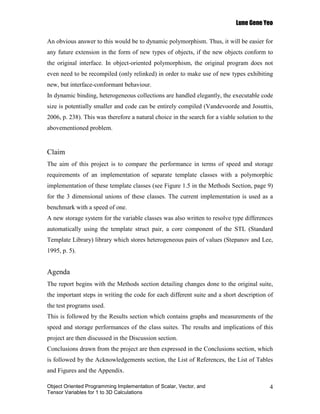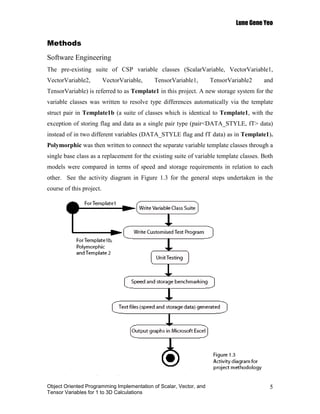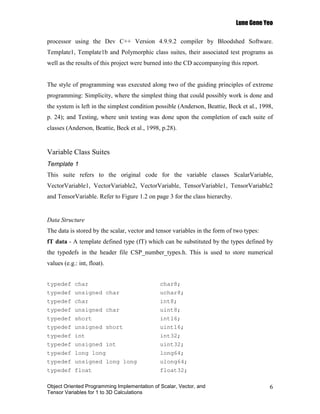Publicité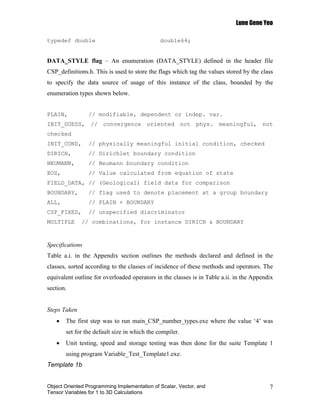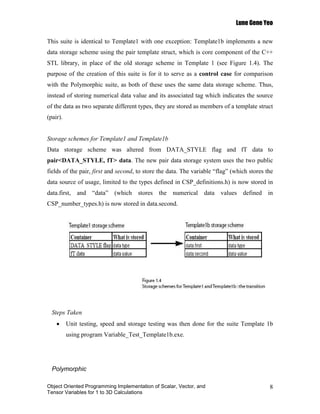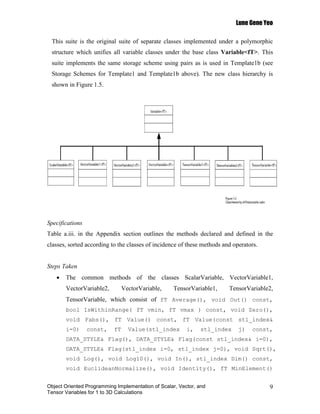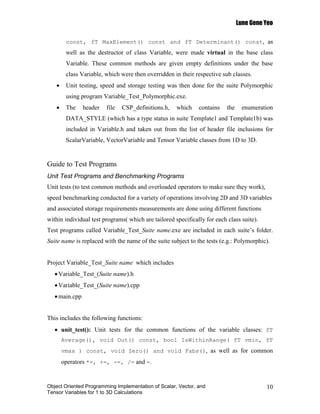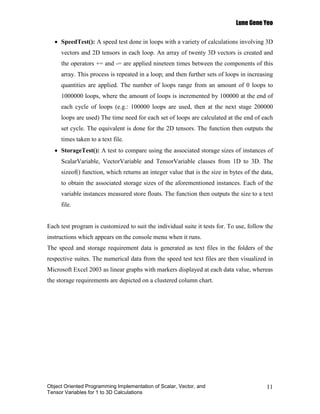Publicité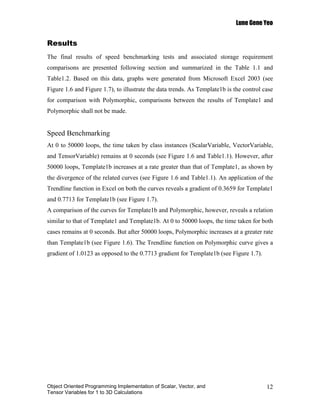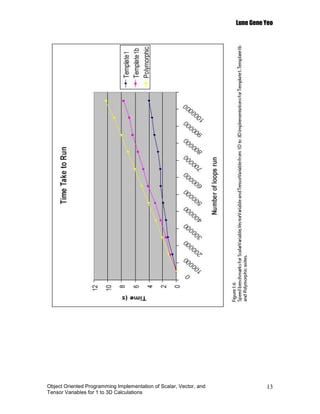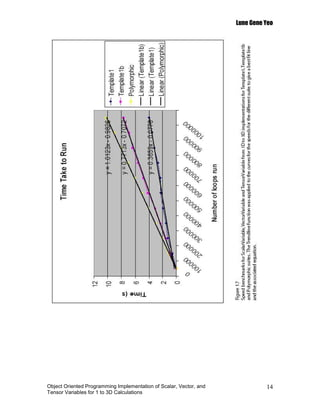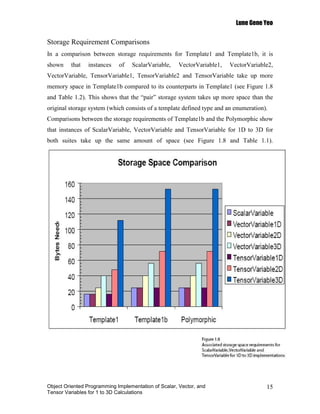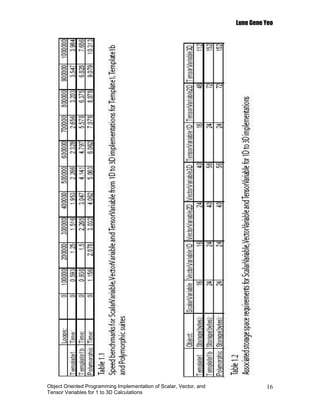Publicité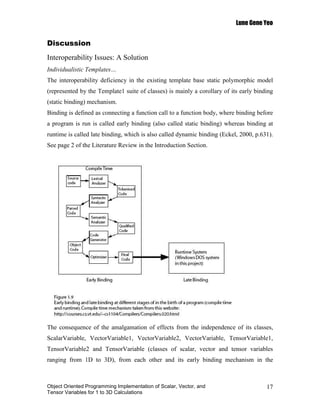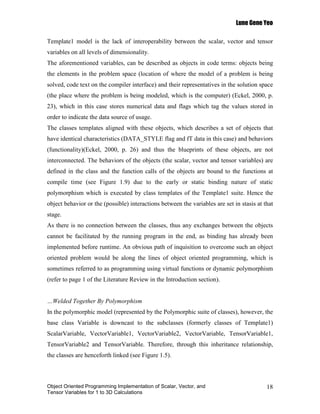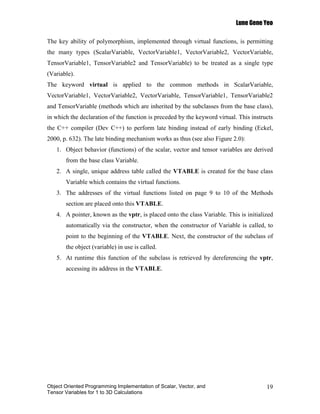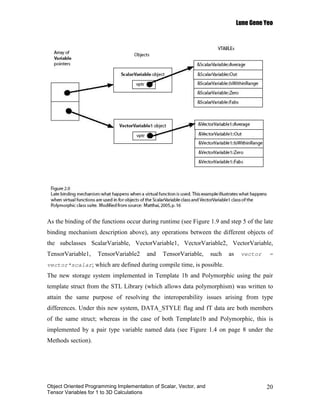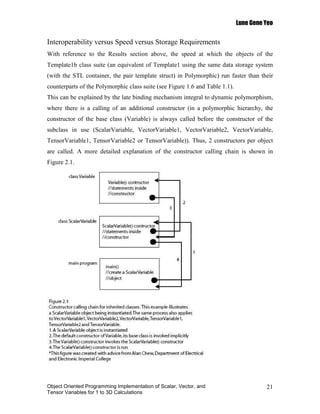Publicité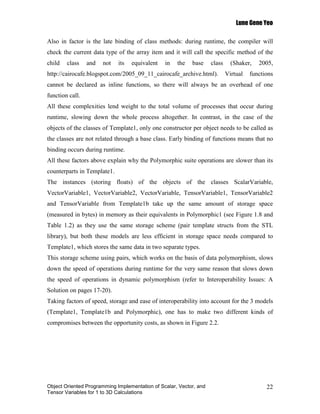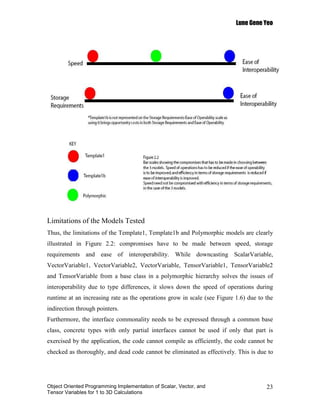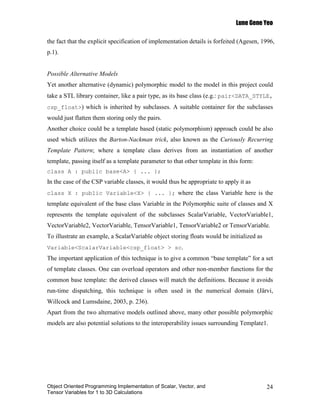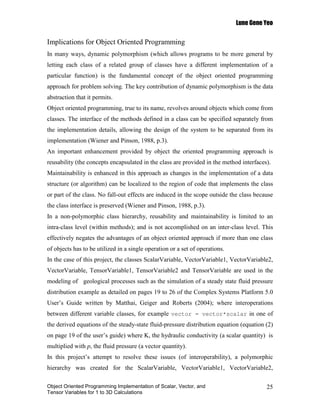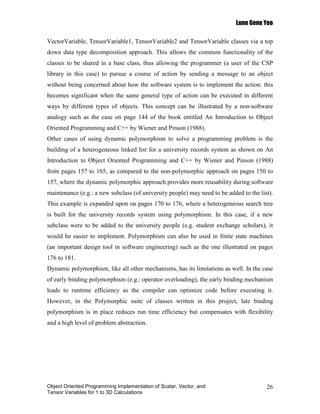Publicité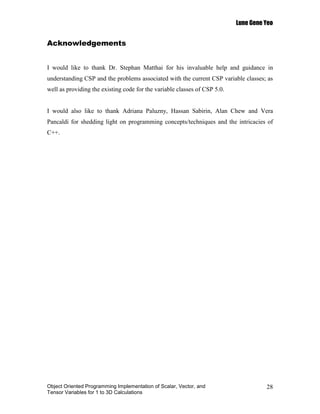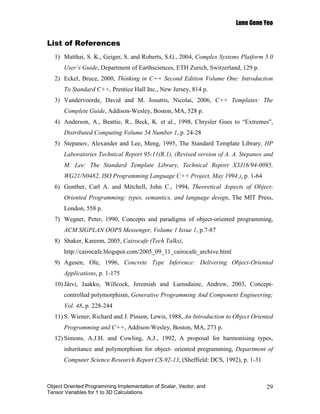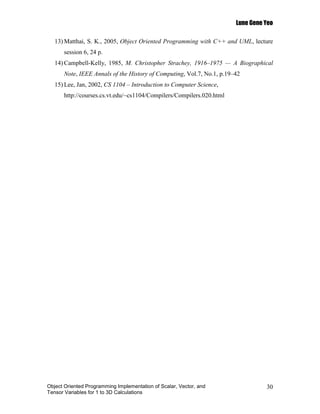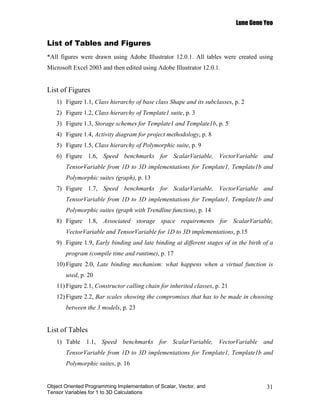Publicité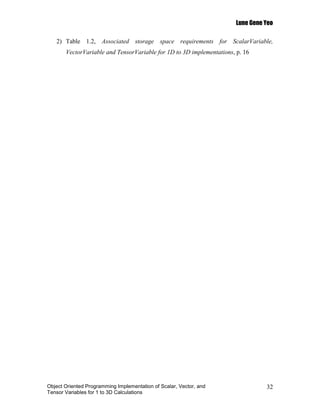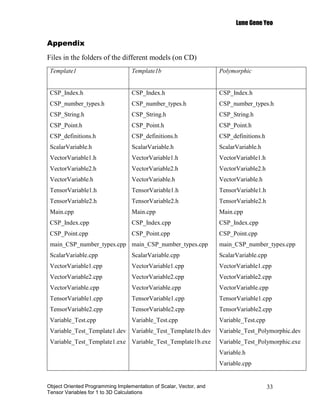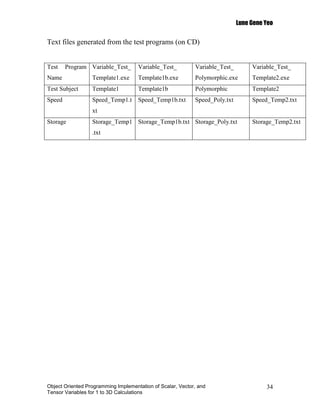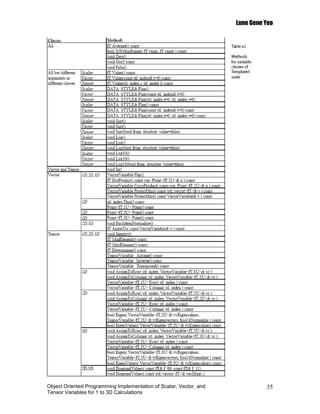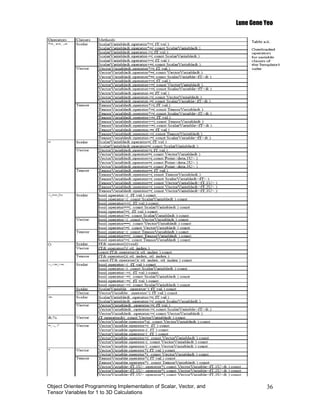Publicité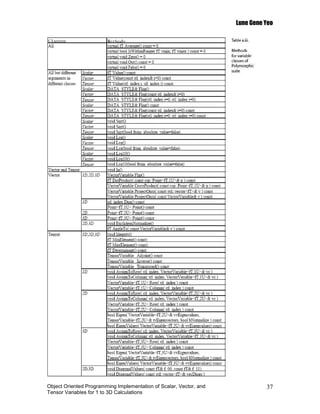Prochain SlideShare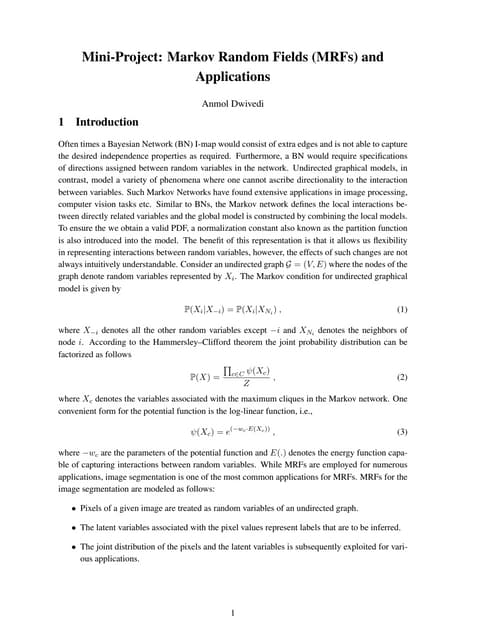Tutorial on Markov Random Fields (MRFs) for Computer Vision Applications
Chargement dans ... 3
1 sur 41
Publicité

### Object Oriented Programming Implementation of Scalar, Vector, and Tensor Variables for 1 to 3D calculations: Comparison Between Polymorphic and Template Based Models (2006)

1. Scalar, Object Oriented Programming Implementation of Scalar, Vector, Tensor Variables calculations: and Tensor Variables for 1 to 3D calculations: Comparison Between Polymorphic and Template Based Models Between Models by Lune Gene Yeo 3rd Year Undergraduate, Department of Earth Science and Engineering, Imperial College A Project Submitted in Fulfillment for the Module 4.09 Independent Project as Partial Fulfillment for the Degree of BSC COMPUTATIONAL GEOSCIENCE Dr. Stephan Matthai, Supervisor (Department of Earth Science and Engineering) ©Lune Gene Yeo, 2006 Imperial College London Submitted on the 19th of February 2007
2. Lune Gene Yeo Abstract Polymorphism is a vital facet of the object oriented programming paradigm. There are five kinds of polymorphism recognized in computer code, two of which are dynamic polymorphism and static polymorphism, which contrast each other in function binding. Dynamic polymorphism utilizes late binding, while static polymorphism uses early binding. In the case of scalar, vector and tensor variables for 1D to 3D calculations in the Complex Systems Platform 5.0 library, a static polymorphic (template) model using individual template classes is in place. This model, however, has issues of interoperability between the different classes of objects. A dynamic polymorphic model was written as an alternative to the first model in an attempt to resolve the aforementioned issues. Results of speed benchmarking and storage comparison tests show that the processes involving the objects from the dynamic polymorphic model run at a slower speed compared to the template model, but both show no difference in storage space required. The properties of the dynamic polymorphic model thus provide an answer to the interoperability issues of the template model at the cost of runtime speed. There are, however, alternative approaches to solving these issues apart from the dynamic polymorphic model written. In retrospect, the reusability of the dynamic polymorphic model has significant implications for software maintenance in the object oriented programming archetype due to its reusability. Keywords CSP, modeling, geological systems, C++, programming, scalar, vector, tensor, polymorphism, templates, static, dynamic, object oriented Object Oriented Programming Implementation of Scalar, Vector, and Tensor Variables for 1 to 3D Calculations
3. Lune Gene Yeo Table of Contents INTRODUCTION………………………………………………………………..1 Literature review…………………………………………………….……1 Claim.……………………………………………………………….…….4 Agenda…………………………………………………….……...............4 METHODS………………………………………………………………….……5 Software Engineering………………………………………………….…5 Variable Class Suites………………………………………………….….6 Guide to Test Programs………………………………………………….10 RESULTS………………………………………………………………………..12 Speed Benchmarking…………………………………………………….12 Storage Requirement Comparisons………………………………………15 DISCUSSION……………………………………………………………………17 Interoperability Issues: A Solution …………………………………...….17 Interoperability versus Speed versus Storage Requirements………..……21 Limitations of the Models Tested ……………………………….….……23 Implications for Object Oriented Programming……………………..…...25 CONCLUSIONS…………………………………………………………..……..27 ACKNOWLEDGEMENTS……………………………………………….…...…28 LIST OF REFERENCES………………………………………………..…….….29 LISTS OF TABLES AND FIGURES…………………………………….…...….31 APPENDIX……………………………………………………………….…...….33 Object Oriented Programming Implementation of Scalar, Vector, and Tensor Variables for 1 to 3D Calculations
4. Lune Gene Yeo Introduction Literature Review What is polymorphism? Polymorphism (in Ancient Greek, poly means “many” and “morph” means form), when defined in non-software terms, literally means the ability to assume many forms. Polymorphism in computer code was first identified by Christopher Strachey, a British computer scientist in his 1967 book Fundamental Concepts of Programming Languages (Campbell-Kelly, 1985, p. 19-42). In the object oriented programming paradigm, polymorphism is described as a feature in which the behavior of a class may be reused in the definition of new classes, according to a paper entitled Concepts and Paradigms of Object-Oriented Programming by Wegner (1990, p. 10). In their paper, A Proposal for Harmonising Types, Inheritance and Polymorphism For Object-Oriented Programming, Simons and Cowling (1992, p.16-17) classify polymorphism into 5 kinds: universal polymorphism, bounded polymorphism, universal construction, bounded construction and arbitrary union. In this project, implementations of two of these kinds are explored and compared, namely bounded polymorphism, also known as dynamic polymorphism (this is the term that will be used throughout the rest of the this report) and universal construction, also known as parametric polymorphism or static polymorphism. Dynamic polymorphism versus static polymorphism When we talk of basic polymorphism, the first assumption would be that we are referring to dynamic polymorphism based on inheritance (Gunther and Mitchell, 1994, p. 497) where several subclasses are connected through a base class through a hierarchical organization of classes; as opposed to static polymorphism which is defined as programming using templates (Vandevoorde and Josuttis, 2006, p.240). A classic example of dynamic polymorphism in application would be a generic class Shape which is inherited by a group of classes: Circle, Square and Triangle. These classes are blueprints for objects representing geometric shapes (Eckel, 2000, p. 39-41) as shown in Figure 1.1. The class Shape declares the common operations and properties for the Circle, Square and Triangle classes: these common operations and properties are defined Object Oriented Programming Implementation of Scalar, Vector, and 1 Tensor Variables for 1 to 3D Calculations
5. Lune Gene Yeo in the individual subclasses themselves. In the base class, common operations are usually declared and defined as virtual functions. Thus, dynamic polymorphism is effectively programming with virtual functions, also thought of as object oriented programming (Vandevoorde and Josuttis, 2006, p. 240). Static polymorphism, when practically applied, is followed by the notion of generic programming which is utilized in the C++ programming language through templates (Vandevoorde and Josuttis, 2006, p. 240); where instead of factoring common behaviour in base classes, the objects from different classes in an application must support operations using a common syntax. The different concrete classes are defined independently form each other. This version of polymorphism defines the intra-class nature of each of the Complex Systems Platform (CSP) 5.0 variable classes (see Figure 1.2). Binding: Early or Late? One question, however, arises when attempting to treat derived object types as their generic base types. If a function of the same name is called while ignoring the specific (derived) type, how will the right behaviour occur? There are two paths to an answer: through static polymorphism, using early binding; or through dynamic polymorphism using late binding. In early (static) binding, the compiler generates a call to a specific function name, and the linker resolves this call to the absolute address of the code to be executed; all of which is done during compile time. In late (dynamic) binding, the code being called is not determined until runtime (Eckel, 2000, p. 37-38). Object Oriented Programming Implementation of Scalar, Vector, and 2 Tensor Variables for 1 to 3D Calculations
6. Lune Gene Yeo Complex Systems Platform (CSP) 5.0 Variables The Complex Systems Platform (CSP) 5.0 is a C++ language based library used for the modeling of geological processes and their interactions, invented by S.K. Matthai in collaboration with S. G. Roberts (ANU), and Sebastian Geiger (ETH) for the PC, Macintosh and UNIX workstations (Matthai, Geiger and Roberts, 2004, p. 1). The CSP variable classes comprise of a ScalarVariable, a VectorVariable and a Tensor Variable template (see Figure 1.2): VectorVariable and TensorVariable have 1, 2 and 3D template specializations which store different types of data (e.g.: float, integer, double) for the purpose of calculations. Each value is tagged with a flag which specifies the data source of usage of this instance of the class. These classes are not connected to each other through a base class or a template equivalent of a base class. The Problems and Possible Solutions There is a key issue that arises from the type differences between the individual CSP scalar, vector and tensor variable static polymorphic template classes as they are declared and defined individually from each other. This is the lack of interoperability between instances of the scalar, vector and tensor classes due to the type differences of the variables which are not derived from a common base class. Therefore one always has to write if statements like: if ( scalar )... else if ( tensor )... Another qualm from this lack of interoperability is that direct applications of operators between different classes like vector = vector*scalar is not possible. The issues outlined above do not only apply for CSP variables, however, but to any range of classes which are used in operations or functions with one another. Object Oriented Programming Implementation of Scalar, Vector, and 3 Tensor Variables for 1 to 3D Calculations
7. Lune Gene Yeo An obvious answer to this would be to dynamic polymorphism. Thus, it will be easier for any future extension in the form of new types of objects, if the new objects conform to the original interface. In object-oriented polymorphism, the original program does not even need to be recompiled (only relinked) in order to make use of new types exhibiting new, but interface-conformant behaviour. In dynamic binding, heterogeneous collections are handled elegantly, the executable code size is potentially smaller and code can be entirely compiled (Vandevoorde and Josuttis, 2006, p. 238). This was therefore a natural choice in the search for a viable solution to the abovementioned problem. Claim The aim of this project is to compare the performance in terms of speed and storage requirements of an implementation of separate template classes with a polymorphic implementation of these template classes (see Figure 1.5 in the Methods Section, page 9) for the 3 dimensional unions of these classes. The current implementation is used as a benchmark with a speed of one. A new storage system for the variable classes was also written to resolve type differences automatically using the template struct pair, a core component of the STL (Standard Template Library) library which stores heterogeneous pairs of values (Stepanov and Lee, 1995, p. 5). Agenda The report begins with the Methods section detailing changes done to the original suite, the important steps in writing the code for each different suite and a short description of the test programs used. This is followed by the Results section which contains graphs and measurements of the speed and storage performances of the class suites. The results and implications of this project are then discussed in the Discussion section. Conclusions drawn from the project are then expressed in the Conclusions section, which is followed by the Acknowledgements section, the List of References, the List of Tables and Figures and the Appendix. Object Oriented Programming Implementation of Scalar, Vector, and 4 Tensor Variables for 1 to 3D Calculations
8. Lune Gene Yeo Methods Software Engineering The pre-existing suite of CSP variable classes (ScalarVariable, VectorVariable1, VectorVariable2, VectorVariable, TensorVariable1, TensorVariable2 and TensorVariable) is referred to as Template1 in this project. A new storage system for the variable classes was written to resolve type differences automatically via the template struct pair in Template1b (a suite of classes which is identical to Template1, with the exception of storing flag and data as a single pair type (pair<DATA_STYLE, fT> data) instead of in two different variables (DATA_STYLE flag and fT data) as in Template1). Polymorphic was then written to connect the separate variable template classes through a single base class as a replacement for the existing suite of variable template classes. Both models were compared in terms of speed and storage requirements in relation to each other. See the activity diagram in Figure 1.3 for the general steps undertaken in the course of this project. All programming was done in the C++ programming language as it supports dynamic binding, and hence polymorphism. The code was compiled on the Intel(R) Pentium(R) M Object Oriented Programming Implementation of Scalar, Vector, and 5 Tensor Variables for 1 to 3D Calculations
9. Lune Gene Yeo processor using the Dev C++ Version 4.9.9.2 compiler by Bloodshed Software. Template1, Template1b and Polymorphic class suites, their associated test programs as well as the results of this project were burned into the CD accompanying this report. The style of programming was executed along two of the guiding principles of extreme programming: Simplicity, where the simplest thing that could possibly work is done and the system is left in the simplest condition possible (Anderson, Beattie, Beck et al., 1998, p. 24); and Testing, where unit testing was done upon the completion of each suite of classes (Anderson, Beattie, Beck et al., 1998, p.28). Variable Class Suites Template 1 This suite refers to the original code for the variable classes ScalarVariable, VectorVariable1, VectorVariable2, VectorVariable, TensorVariable1, TensorVariable2 and TensorVariable. Refer to Figure 1.2 on page 3 for the class hierarchy. Data Structure The data is stored by the scalar, vector and tensor variables in the form of two types: fT data - A template defined type (fT) which can be substituted by the types defined by the typedefs in the header file CSP_number_types.h. This is used to store numerical values (e.g.: int, float). typedef char char8; typedef unsigned char uchar8; typedef char int8; typedef unsigned char uint8; typedef short int16; typedef unsigned short uint16; typedef int int32; typedef unsigned int uint32; typedef long long long64; typedef unsigned long long ulong64; typedef float float32; Object Oriented Programming Implementation of Scalar, Vector, and 6 Tensor Variables for 1 to 3D Calculations
10. Lune Gene Yeo typedef double double64; DATA_STYLE flag – An enumeration (DATA_STYLE) defined in the header file CSP_definitions.h. This is used to store the flags which tag the values stored by the class to specify the data source of usage of this instance of the class, bounded by the enumeration types shown below. PLAIN, // modifiable, dependent or indep. var. INIT_GUESS, // convergence oriented not phys. meaningful, not checked INIT_COND, // physically meaningful initial condition, checked DIRICH, // Dirichlet boundary condition NEUMANN, // Neumann boundary condition EOS, // Value calculated from equation of state FIELD_DATA, // (Geological) field data for comparison BOUNDARY, // flag used to denote placement at a group boundary ALL, // PLAIN + BOUNDARY CSP_FIXED, // unspecified discriminator MULTIPLE // combinations, for instance DIRICH & BOUNDARY Specifications Table a.i. in the Appendix section outlines the methods declared and defined in the classes, sorted according to the classes of incidence of these methods and operators. The equivalent outline for overloaded operators in the classes is in Table a.ii. in the Appendix section. Steps Taken • The first step was to run main_CSP_number_types.exe where the value ‘4’ was set for the default size in which the compiler. • Unit testing, speed and storage testing was then done for the suite Template 1 using program Variable_Test_Template1.exe. Template 1b Object Oriented Programming Implementation of Scalar, Vector, and 7 Tensor Variables for 1 to 3D Calculations
11. Lune Gene Yeo This suite is identical to Template1 with one exception: Template1b implements a new data storage scheme using the pair template struct, which is core component of the C++ STL library, in place of the old storage scheme in Template 1 (see Figure 1.4). The purpose of the creation of this suite is for it to serve as a control case for comparison with the Polymorphic suite, as both of these uses the same data storage scheme. Thus, instead of storing numerical data value and its associated tag which indicates the source of the data as two separate different types, they are stored as members of a template struct (pair). Storage schemes for Template1 and Template1b Data storage scheme was altered from DATA_STYLE flag and fT data to pair<DATA_STYLE, fT> data. The new pair data storage system uses the two public fields of the pair, first and second, to store the data. The variable “flag” (which stores the data source of usage, limited to the types defined in CSP_definitions.h) is now stored in data.first, and “data” (which stores the numerical data values defined in CSP_number_types.h) is now stored in data.second. Steps Taken • Unit testing, speed and storage testing was then done for the suite Template 1b using program Variable_Test_Template1b.exe. Polymorphic Object Oriented Programming Implementation of Scalar, Vector, and 8 Tensor Variables for 1 to 3D Calculations
12. Lune Gene Yeo This suite is the original suite of separate classes implemented under a polymorphic structure which unifies all variable classes under the base class Variable<fT>. This suite implements the same storage scheme using pairs as is used in Template1b (see Storage Schemes for Template1 and Template1b above). The new class hierarchy is shown in Figure 1.5. Specifications Table a.iii. in the Appendix section outlines the methods declared and defined in the classes, sorted according to the classes of incidence of these methods and operators. Steps Taken • The common methods of the classes ScalarVariable, VectorVariable1, VectorVariable2, VectorVariable, TensorVariable1, TensorVariable2, TensorVariable, which consist of fT Average(), void Out() const, bool IsWithinRange( fT vmin, fT vmax ) const, void Zero(), void Fabs(), fT Value() const, fT Value(const stl_index& i=0) const, fT Value(stl_index i, stl_index j) const, DATA_STYLE& Flag(), DATA_STYLE& Flag(const stl_index& i=0), DATA_STYLE& Flag(stl_index i=0, stl_index j=0), void Sqrt(), void Log(), void Log10(), void In(), stl_index Dim() const, void EuclideanNormalize(), void Identity(), fT MinElement() Object Oriented Programming Implementation of Scalar, Vector, and 9 Tensor Variables for 1 to 3D Calculations
13. Lune Gene Yeo const, fT MaxElement() const and fT Determinant() const, as well as the destructor of class Variable, were made virtual in the base class Variable. These common methods are given empty definitions under the base class Variable, which were then overridden in their respective sub classes. • Unit testing, speed and storage testing was then done for the suite Polymorphic using program Variable_Test_Polymorphic.exe. • The header file CSP_definitions.h, which contains the enumeration DATA_STYLE (which has a type status in suite Template1 and Template1b) was included in Variable.h and taken out from the list of header file inclusions for ScalarVariable, VectorVariable and Tensor Variable classes from 1D to 3D. Guide to Test Programs Unit Test Programs and Benchmarking Programs Unit tests (to test common methods and overloaded operators to make sure they work), speed benchmarking conducted for a variety of operations involving 2D and 3D variables and associated storage requirements measurements are done using different functions within individual test programs( which are tailored specifically for each class suite). Test programs called Variable_Test_Suite name.exe are included in each suite’s folder. Suite name is replaced with the name of the suite subject to the tests (e.g.: Polymorphic). Project Variable_Test_Suite name which includes • Variable_Test_(Suite name).h • Variable_Test_(Suite name).cpp • main.cpp This includes the following functions: • unit_test(): Unit tests for the common functions of the variable classes: fT Average(), void Out() const, bool IsWithinRange( fT vmin, fT vmax ) const, void Zero() and void Fabs(), as well as for common operators *=, +=, -=, /= and =. Object Oriented Programming Implementation of Scalar, Vector, and 10 Tensor Variables for 1 to 3D Calculations
14. Lune Gene Yeo • SpeedTest(): A speed test done in loops with a variety of calculations involving 3D vectors and 2D tensors in each loop. An array of twenty 3D vectors is created and the operators += and -= are applied nineteen times between the components of this array. This process is repeated in a loop; and then further sets of loops in increasing quantities are applied. The number of loops range from an amount of 0 loops to 1000000 loops, where the amount of loops is incremented by 100000 at the end of each cycle of loops (e.g.: 100000 loops are used, then at the next stage 200000 loops are used) The time need for each set of loops are calculated at the end of each set cycle. The equivalent is done for the 2D tensors. The function then outputs the times taken to a text file. • StorageTest(): A test to compare using the associated storage sizes of instances of ScalarVariable, VectorVariable and TensorVariable classes from 1D to 3D. The sizeof() function, which returns an integer value that is the size in bytes of the data, to obtain the associated storage sizes of the aforementioned instances. Each of the variable instances measured store floats. The function then outputs the size to a text file. Each test program is customized to suit the individual suite it tests for. To use, follow the instructions which appears on the console menu when it runs. The speed and storage requirement data is generated as text files in the folders of the respective suites. The numerical data from the speed test text files are then visualized in Microsoft Excel 2003 as linear graphs with markers displayed at each data value, whereas the storage requirements are depicted on a clustered column chart. Object Oriented Programming Implementation of Scalar, Vector, and 11 Tensor Variables for 1 to 3D Calculations
15. Lune Gene Yeo Results The final results of speed benchmarking tests and associated storage requirement comparisons are presented following section and summarized in the Table 1.1 and Table1.2. Based on this data, graphs were generated from Microsoft Excel 2003 (see Figure 1.6 and Figure 1.7), to illustrate the data trends. As Template1b is the control case for comparison with Polymorphic, comparisons between the results of Template1 and Polymorphic shall not be made. Speed Benchmarking At 0 to 50000 loops, the time taken by class instances (ScalarVariable, VectorVariable, and TensorVariable) remains at 0 seconds (see Figure 1.6 and Table1.1). However, after 50000 loops, Template1b increases at a rate greater than that of Template1, as shown by the divergence of the related curves (see Figure 1.6 and Table1.1). An application of the Trendline function in Excel on both the curves reveals a gradient of 0.3659 for Template1 and 0.7713 for Template1b (see Figure 1.7). A comparison of the curves for Template1b and Polymorphic, however, reveals a relation similar to that of Template1 and Template1b. At 0 to 50000 loops, the time taken for both cases remains at 0 seconds. But after 50000 loops, Polymorphic increases at a greater rate than Template1b (see Figure 1.6). The Trendline function on Polymorphic curve gives a gradient of 1.0123 as opposed to the 0.7713 gradient for Template1b (see Figure 1.7). Object Oriented Programming Implementation of Scalar, Vector, and 12 Tensor Variables for 1 to 3D Calculations
16. Lune Gene Yeo Object Oriented Programming Implementation of Scalar, Vector, and 13 Tensor Variables for 1 to 3D Calculations
17. Lune Gene Yeo Object Oriented Programming Implementation of Scalar, Vector, and 14 Tensor Variables for 1 to 3D Calculations
18. Lune Gene Yeo Storage Requirement Comparisons In a comparison between storage requirements for Template1 and Template1b, it is shown that instances of ScalarVariable, VectorVariable1, VectorVariable2, VectorVariable, TensorVariable1, TensorVariable2 and TensorVariable take up more memory space in Template1b compared to its counterparts in Template1 (see Figure 1.8 and Table 1.2). This shows that the “pair” storage system takes up more space than the original storage system (which consists of a template defined type and an enumeration). Comparisons between the storage requirements of Template1b and the Polymorphic show that instances of ScalarVariable, VectorVariable and TensorVariable for 1D to 3D for both suites take up the same amount of space (see Figure 1.8 and Table 1.1). Object Oriented Programming Implementation of Scalar, Vector, and 15 Tensor Variables for 1 to 3D Calculations
19. Lune Gene Yeo Object Oriented Programming Implementation of Scalar, Vector, and 16 Tensor Variables for 1 to 3D Calculations
20. Lune Gene Yeo Discussion Interoperability Issues: A Solution Individualistic Templates… The interoperability deficiency in the existing template base static polymorphic model (represented by the Template1 suite of classes) is mainly a corollary of its early binding (static binding) mechanism. Binding is defined as connecting a function call to a function body, where binding before a program is run is called early binding (also called static binding) whereas binding at runtime is called late binding, which is also called dynamic binding (Eckel, 2000, p.631). See page 2 of the Literature Review in the Introduction Section. The consequence of the amalgamation of effects from the independence of its classes, ScalarVariable, VectorVariable1, VectorVariable2, VectorVariable, TensorVariable1, TensorVariable2 and TensorVariable (classes of scalar, vector and tensor variables ranging from 1D to 3D), from each other and its early binding mechanism in the Object Oriented Programming Implementation of Scalar, Vector, and 17 Tensor Variables for 1 to 3D Calculations
21. Lune Gene Yeo Template1 model is the lack of interoperability between the scalar, vector and tensor variables on all levels of dimensionality. The aforementioned variables, can be described as objects in code terms: objects being the elements in the problem space (location of where the model of a problem is being solved, code text on the compiler interface) and their representatives in the solution space (the place where the problem is being modeled, which is the computer) (Eckel, 2000, p. 23), which in this case stores numerical data and flags which tag the values stored in order to indicate the data source of usage. The classes templates aligned with these objects, which describes a set of objects that have identical characteristics (DATA_STYLE flag and fT data in this case) and behaviors (functionality)(Eckel, 2000, p. 26) and thus the blueprints of these objects, are not interconnected. The behaviors of the objects (the scalar, vector and tensor variables) are defined in the class and the function calls of the objects are bound to the functions at compile time (see Figure 1.9) due to the early or static binding nature of static polymorphism which is executed by class templates of the Template1 suite. Hence the object behavior or the (possible) interactions between the variables are set in stasis at that stage. As there is no connection between the classes, thus any exchanges between the objects cannot be facilitated by the running program in the end, as binding has already been implemented before runtime. An obvious path of inquisition to overcome such an object oriented problem would be along the lines of object oriented programming, which is sometimes referred to as programming using virtual functions or dynamic polymorphism (refer to page 1 of the Literature Review in the Introduction section). …Welded Together By Polymorphism In the polymorphic model (represented by the Polymorphic suite of classes), however, the base class Variable is downcast to the subclasses (formerly classes of Template1) ScalarVariable, VectorVariable1, VectorVariable2, VectorVariable, TensorVariable1, TensorVariable2 and TensorVariable. Therefore, through this inheritance relationship, the classes are henceforth linked (see Figure 1.5). Object Oriented Programming Implementation of Scalar, Vector, and 18 Tensor Variables for 1 to 3D Calculations
22. Lune Gene Yeo The key ability of polymorphism, implemented through virtual functions, is permitting the many types (ScalarVariable, VectorVariable1, VectorVariable2, VectorVariable, TensorVariable1, TensorVariable2 and TensorVariable) to be treated as a single type (Variable). The keyword virtual is applied to the common methods in ScalarVariable, VectorVariable1, VectorVariable2, VectorVariable, TensorVariable1, TensorVariable2 and TensorVariable (methods which are inherited by the subclasses from the base class), in which the declaration of the function is preceded by the keyword virtual. This instructs the C++ compiler (Dev C++) to perform late binding instead of early binding (Eckel, 2000, p. 632). The late binding mechanism works as thus (see also Figure 2.0): 1. Object behavior (functions) of the scalar, vector and tensor variables are derived from the base class Variable. 2. A single, unique address table called the VTABLE is created for the base class Variable which contains the virtual functions. 3. The addresses of the virtual functions listed on page 9 to 10 of the Methods section are placed onto this VTABLE. 4. A pointer, known as the vptr, is placed onto the class Variable. This is initialized automatically via the constructor, when the constructor of Variable is called, to point to the beginning of the VTABLE. Next, the constructor of the subclass of the object (variable) in use is called. 5. At runtime this function of the subclass is retrieved by dereferencing the vptr, accessing its address in the VTABLE. Object Oriented Programming Implementation of Scalar, Vector, and 19 Tensor Variables for 1 to 3D Calculations
23. Lune Gene Yeo As the binding of the functions occur during runtime (see Figure 1.9 and step 5 of the late binding mechanism description above), any operations between the different objects of the subclasses ScalarVariable, VectorVariable1, VectorVariable2, VectorVariable, TensorVariable1, TensorVariable2 and TensorVariable, such as vector = vector*scalar; which are defined during compile time, is possible. The new storage system implemented in Template 1b and Polymorphic using the pair template struct from the STL Library (which allows data polymorphism) was written to attain the same purpose of resolving the interoperability issues arising from type differences. Under this new system, DATA_STYLE flag and fT data are both members of the same struct; whereas in the case of both Template1b and Polymorphic, this is implemented by a pair type variable named data (see Figure 1.4 on page 8 under the Methods section). Object Oriented Programming Implementation of Scalar, Vector, and 20 Tensor Variables for 1 to 3D Calculations
24. Lune Gene Yeo Interoperability versus Speed versus Storage Requirements With reference to the Results section above, the speed at which the objects of the Template1b class suite (an equivalent of Template1 using the same data storage system (with the STL container, the pair template struct) in Polymorphic) run faster than their counterparts of the Polymorphic class suite (see Figure 1.6 and Table 1.1). This can be explained by the late binding mechanism integral to dynamic polymorphism, where there is a calling of an additional constructor (in a polymorphic hierarchy, the constructor of the base class (Variable) is always called before the constructor of the subclass in use (ScalarVariable, VectorVariable1, VectorVariable2, VectorVariable, TensorVariable1, TensorVariable2 or TensorVariable)). Thus, 2 constructors per object are called. A more detailed explanation of the constructor calling chain is shown in Figure 2.1. Object Oriented Programming Implementation of Scalar, Vector, and 21 Tensor Variables for 1 to 3D Calculations
25. Lune Gene Yeo Also in factor is the late binding of class methods: during runtime, the compiler will check the current data type of the array item and it will call the specific method of the child class and not its equivalent in the base class (Shaker, 2005, http://cairocafe.blogspot.com/2005_09_11_cairocafe_archive.html). Virtual functions cannot be declared as inline functions, so there will always be an overhead of one function call. All these complexities lend weight to the total volume of processes that occur during runtime, slowing down the whole process altogether. In contrast, in the case of the objects of the classes of Template1, only one constructor per object needs to be called as the classes are not related through a base class. Early binding of functions means that no binding occurs during runtime. All these factors above explain why the Polymorphic suite operations are slower than its counterparts in Template1. The instances (storing floats) of the objects of the classes ScalarVariable, VectorVariable1, VectorVariable2, VectorVariable, TensorVariable1, TensorVariable2 and TensorVariable from Template1b take up the same amount of storage space (measured in bytes) in memory as their equivalents in Polymorphic1 (see Figure 1.8 and Table 1.2) as they use the same storage scheme (pair template structs from the STL library), but both these models are less efficient in storage space needs compared to Template1, which stores the same data in two separate types. This storage scheme using pairs, which works on the basis of data polymorphism, slows down the speed of operations during runtime for the very same reason that slows down the speed of operations in dynamic polymorphism (refer to Interoperability Issues: A Solution on pages 17-20). Taking factors of speed, storage and ease of interoperability into account for the 3 models (Template1, Template1b and Polymorphic), one has to make two different kinds of compromises between the opportunity costs, as shown in Figure 2.2. Object Oriented Programming Implementation of Scalar, Vector, and 22 Tensor Variables for 1 to 3D Calculations
26. Lune Gene Yeo Limitations of the Models Tested Thus, the limitations of the Template1, Template1b and Polymorphic models are clearly illustrated in Figure 2.2: compromises have to be made between speed, storage requirements and ease of interoperability. While downcasting ScalarVariable, VectorVariable1, VectorVariable2, VectorVariable, TensorVariable1, TensorVariable2 and TensorVariable from a base class in a polymorphic hierarchy solves the issues of interoperability due to type differences, it slows down the speed of operations during runtime at an increasing rate as the operations grow in scale (see Figure 1.6) due to the indirection through pointers. Furthermore, the interface commonality needs to be expressed through a common base class, concrete types with only partial interfaces cannot be used if only that part is exercised by the application, the code cannot compile as efficiently, the code cannot be checked as thoroughly, and dead code cannot be eliminated as effectively. This is due to Object Oriented Programming Implementation of Scalar, Vector, and 23 Tensor Variables for 1 to 3D Calculations
27. Lune Gene Yeo the fact that the explicit specification of implementation details is forfeited (Agesen, 1996, p.1). Possible Alternative Models Yet another alternative (dynamic) polymorphic model to the model in this project could take a STL library container, like a pair type, as its base class (e.g.: pair<DATA_STYLE, csp_float>) which is inherited by subclasses. A suitable container for the subclasses would just flatten them storing only the pairs. Another choice could be a template based (static polymorphism) approach could be also used which utilizes the Barton-Nackman trick, also known as the Curiously Recurring Template Pattern; where a template class derives from an instantiation of another template, passing itself as a template parameter to that other template in this form: class A : public base<A> { ... }; In the case of the CSP variable classes, it would thus be appropriate to apply it as class X : public Variable<X> { ... }; where the class Variable here is the template equivalent of the base class Variable in the Polymorphic suite of classes and X represents the template equivalent of the subclasses ScalarVariable, VectorVariable1, VectorVariable2, VectorVariable, TensorVariable1, TensorVariable2 or TensorVariable. To illustrate an example, a ScalarVariable object storing floats would be initialized as Variable<ScalarVariable<csp_float> > sc. The important application of this technique is to give a common “base template” for a set of template classes. One can overload operators and other non-member functions for the common base template: the derived classes will match the definitions. Because it avoids run-time dispatching, this technique is often used in the numerical domain (Järvi, Willcock and Lumsdaine, 2003, p. 236). Apart from the two alternative models outlined above, many other possible polymorphic models are also potential solutions to the interoperability issues surrounding Template1. Object Oriented Programming Implementation of Scalar, Vector, and 24 Tensor Variables for 1 to 3D Calculations
28. Lune Gene Yeo Implications for Object Oriented Programming In many ways, dynamic polymorphism (which allows programs to be more general by letting each class of a related group of classes have a different implementation of a particular function) is the fundamental concept of the object oriented programming approach for problem solving. The key contribution of dynamic polymorphism is the data abstraction that it permits. Object oriented programming, true to its name, revolves around objects which come from classes. The interface of the methods defined in a class can be specified separately from the implementation details, allowing the design of the system to be separated from its implementation (Wiener and Pinson, 1988, p.3). An important enhancement provided by object the oriented programming approach is reusability (the concepts encapsulated in the class are provided in the method interfaces). Maintainability is enhanced in this approach as changes in the implementation of a data structure (or algorithm) can be localized to the region of code that implements the class or part of the class. No fall-out effects are induced in the scope outside the class because the class interface is preserved (Wiener and Pinson, 1988, p.3). In a non-polymorphic class hierarchy, reusability and maintainability is limited to an intra-class level (within methods); and is not accomplished on an inter-class level. This effectively negates the advantages of an object oriented approach if more than one class of objects has to be utilized in a single operation or a set of operations. In the case of this project, the classes ScalarVariable, VectorVariable1, VectorVariable2, VectorVariable, TensorVariable1, TensorVariable2 and TensorVariable are used in the modeling of geological processes such as the simulation of a steady state fluid pressure distribution example as detailed on pages 19 to 26 of the Complex Systems Platform 5.0 User’s Guide written by Matthai, Geiger and Roberts (2004); where interoperations between different variable classes, for example vector = vector*scalar in one of the derived equations of the steady-state fluid-pressure distribution equation (equation (2) on page 19 of the user’s guide) where K, the hydraulic conductivity (a scalar quantity) is multiplied with p, the fluid pressure (a vector quantity). In this project’s attempt to resolve these issues (of interoperability), a polymorphic hierarchy was created for the ScalarVariable, VectorVariable1, VectorVariable2, Object Oriented Programming Implementation of Scalar, Vector, and 25 Tensor Variables for 1 to 3D Calculations
29. Lune Gene Yeo VectorVariable, TensorVariable1, TensorVariable2 and TensorVariable classes via a top down data type decomposition approach. This allows the common functionality of the classes to be shared in a base class, thus allowing the programmer (a user of the CSP library in this case) to pursue a course of action by sending a message to an object without being concerned about how the software system is to implement the action: this becomes significant when the same general type of action can be executed in different ways by different types of objects. This concept can be illustrated by a non-software analogy such as the case on page 144 of the book entitled An Introduction to Object Oriented Programming and C++ by Wiener and Pinson (1988). Other cases of using dynamic polymorphism to solve a programming problem is the building of a heterogeneous linked list for a university records system as shown on An Introduction to Object Oriented Programming and C++ by Wiener and Pinson (1988) from pages 157 to 165, as compared to the non-polymorphic approach on pages 150 to 157, where the dynamic polymorphic approach provides more reusability during software maintenance (e.g.: a new subclass (of university people) may need to be added to the list). This example is expanded upon on pages 170 to 176, where a heterogeneous search tree is built for the university records system using polymorphism. In this case, if a new subclass were to be added to the university people (e.g. student exchange scholars), it would be easier to implement. Polymorphism can also be used in finite state machines (an important design tool in software engineering) such as the one illustrated on pages 176 to 181. Dynamic polymorphism, like all other mechanisms, has its limitations as well. In the case of early binding polymorphism (e.g.: operator overloading), the early binding mechanism leads to runtime efficiency as the compiler can optimize code before executing it. However, in the Polymorphic suite of classes written in this project, late binding polymorphism is in place reduces run time efficiency but compensates with flexibility and a high level of problem abstraction. Object Oriented Programming Implementation of Scalar, Vector, and 26 Tensor Variables for 1 to 3D Calculations
30. Lune Gene Yeo Conclusions The existence of classes which are separate from one another (not connected through a base class or a template equivalent) creates interoperability issues in a situation when the objects of these classes need to be used in operations involving each other. The CSP template (static polymorphic) classes ScalarVariable, VectorVariable1, VectorVariable2, VectorVariable, TensorVariable1, TensorVariable2 and TensorVariable; which are used in tandem with each other to model geological processes and their interactions, provide an excellent example of where these issues may arise. Dynamic polymorphism, or more specifically its inheritance mechanism, was used in an attempt to engineer a solution to these issues through the creation of a new suite of classes, fittingly named Polymorphic. This new model connects the aforementioned CSP template classes through the base class Variable, solving the issues outlined above. However, late binding is a core mechanism of the dynamic polymorphic model, which slows down runtime speed significantly through the added complexity due to the indirection of pointers. A new storage system where the stored data is connected through static polymorphism (with a STL container, pair, which is a template struct) was also implemented in an effort towards solving the same issues involving type difference. This new storage scheme is, however, more inefficient in terms of storage requirements; in addition to also having the same outcome (as dynamic polymorphism) of slowing down runtime processes. Dynamic polymorphism is a crucial element of object oriented programming, having applications in other problems outside the CSP scalar, vector and tensor template classes (1D to 3D) dilemma. Some of these are linked lists, search tress and finite state machines. Other models are possible however, including using an approach based upon the “Barton- Nackman trick” (Curiously Recurring Template Pattern) where a template (static polymorphic) version of the base class in the Polymorphic model can be used to unify the scalar, vector and tensor classes from 1D to 3D. Object Oriented Programming Implementation of Scalar, Vector, and 27 Tensor Variables for 1 to 3D Calculations
31. Lune Gene Yeo Acknowledgements I would like to thank Dr. Stephan Matthai for his invaluable help and guidance in understanding CSP and the problems associated with the current CSP variable classes; as well as providing the existing code for the variable classes of CSP 5.0. I would also like to thank Adriana Paluzny, Hassan Sabirin, Alan Chew and Vera Pancaldi for shedding light on programming concepts/techniques and the intricacies of C++. Object Oriented Programming Implementation of Scalar, Vector, and 28 Tensor Variables for 1 to 3D Calculations
32. Lune Gene Yeo List of References 1) Matthai, S. K., Geiger, S. and Roberts, S.G., 2004, Complex Systems Platform 5.0 User’s Guide, Department of Earthsciences, ETH Zurich, Switzerland, 129 p. 2) Eckel, Bruce, 2000, Thinking in C++ Second Edition Volume One: Introduction To Standard C++, Prentice Hall Inc., New Jersey, 814 p. 3) Vandervoorde, David and M. Josuttis, Nicolai, 2006, C++ Templates: The Complete Guide, Addison-Wesley, Boston, MA, 528 p. 4) Anderson, A., Beattie, R., Beck, K. et al., 1998, Chrysler Goes to “Extremes", Distributed Computing Volume 54 Number 1, p. 24-28 5) Stepanov, Alexander and Lee, Meng, 1995, The Standard Template Library, HP Laboratories Technical Report 95-11(R.1), (Revised version of A. A. Stepanov and M. Lee: The Standard Template Library, Technical Report X3J16/94-0095, WG21/N0482, ISO Programming Language C++ Project, May 1994.), p. 1-64 6) Gunther, Carl A. and Mitchell, John C., 1994, Theoretical Aspects of Object- Oriented Programming: types, semantics, and language design, The MIT Press, London, 558 p. 7) Wegner, Peter, 1990, Concepts and paradigms of object-oriented programming, ACM SIGPLAN OOPS Messenger, Volume 1 Issue 1, p.7-87 8) Shaker, Kareem, 2005, Cairocafe (Tech Talks), http://cairocafe.blogspot.com/2005_09_11_cairocafe_archive.html 9) Agesen, Ole, 1996, Concrete Type Inference: Delivering Object-Oriented Applications, p. 1-175 10) Järvi, Jaakko, Willcock, Jeremiah and Lumsdaine, Andrew, 2003, Concept- controlled polymorphism, Generative Programming And Component Engineering; Vol. 48, p. 228-244 11) S. Wiener, Richard and J. Pinson, Lewis, 1988, An Introduction to Object Oriented Programming and C++, Addison-Wesley, Boston, MA, 273 p. 12) Simons, A.J.H. and Cowling, A.J., 1992, A proposal for harmonising types, inheritance and polymorphism for object- oriented programming, Department of Computer Science Research Report CS-92-13, (Sheffield: DCS, 1992), p. 1-31 Object Oriented Programming Implementation of Scalar, Vector, and 29 Tensor Variables for 1 to 3D Calculations
33. Lune Gene Yeo 13) Matthai, S. K., 2005, Object Oriented Programming with C++ and UML, lecture session 6, 24 p. 14) Campbell-Kelly, 1985, M. Christopher Strachey, 1916–1975 — A Biographical Note, IEEE Annals of the History of Computing, Vol.7, No.1, p.19–42 15) Lee, Jan, 2002, CS 1104 – Introduction to Computer Science, http://courses.cs.vt.edu/~cs1104/Compilers/Compilers.020.html Object Oriented Programming Implementation of Scalar, Vector, and 30 Tensor Variables for 1 to 3D Calculations
34. Lune Gene Yeo List of Tables and Figures *All figures were drawn using Adobe Illustrator 12.0.1. All tables were created using Microsoft Excel 2003 and then edited using Adobe Illustrator 12.0.1. List of Figures 1) Figure 1.1, Class hierarchy of base class Shape and its subclasses, p. 2 2) Figure 1.2, Class hierarchy of Template1 suite, p. 3 3) Figure 1.3, Storage schemes for Template1 and Template1b, p. 5 4) Figure 1.4, Activity diagram for project methodology, p. 8 5) Figure 1.5, Class hierarchy of Polymorphic suite, p. 9 6) Figure 1.6, Speed benchmarks for ScalarVariable, VectorVariable and TensorVariable from 1D to 3D implementations for Template1, Template1b and Polymorphic suites (graph), p. 13 7) Figure 1.7, Speed benchmarks for ScalarVariable, VectorVariable and TensorVariable from 1D to 3D implementations for Template1, Template1b and Polymorphic suites (graph with Trendline function), p. 14 8) Figure 1.8, Associated storage space requirements for ScalarVariable, VectorVariable and TensorVariable for 1D to 3D implementations, p.15 9) Figure 1.9, Early binding and late binding at different stages of in the birth of a program (compile time and runtime), p. 17 10) Figure 2.0, Late binding mechanism: what happens when a virtual function is used, p. 20 11) Figure 2.1, Constructor calling chain for inherited classes, p. 21 12) Figure 2.2, Bar scales showing the compromises that has to be made in choosing between the 3 models, p. 23 List of Tables 1) Table 1.1, Speed benchmarks for ScalarVariable, VectorVariable and TensorVariable from 1D to 3D implementations for Template1, Template1b and Polymorphic suites, p. 16 Object Oriented Programming Implementation of Scalar, Vector, and 31 Tensor Variables for 1 to 3D Calculations
35. Lune Gene Yeo 2) Table 1.2, Associated storage space requirements for ScalarVariable, VectorVariable and TensorVariable for 1D to 3D implementations, p. 16 Object Oriented Programming Implementation of Scalar, Vector, and 32 Tensor Variables for 1 to 3D Calculations
36. Lune Gene Yeo Appendix Files in the folders of the different models (on CD) Template1 Template1b Polymorphic CSP_Index.h CSP_Index.h CSP_Index.h CSP_number_types.h CSP_number_types.h CSP_number_types.h CSP_String.h CSP_String.h CSP_String.h CSP_Point.h CSP_Point.h CSP_Point.h CSP_definitions.h CSP_definitions.h CSP_definitions.h ScalarVariable.h ScalarVariable.h ScalarVariable.h VectorVariable1.h VectorVariable1.h VectorVariable1.h VectorVariable2.h VectorVariable2.h VectorVariable2.h VectorVariable.h VectorVariable.h VectorVariable.h TensorVariable1.h TensorVariable1.h TensorVariable1.h TensorVariable2.h TensorVariable2.h TensorVariable2.h Main.cpp Main.cpp Main.cpp CSP_Index.cpp CSP_Index.cpp CSP_Index.cpp CSP_Point.cpp CSP_Point.cpp CSP_Point.cpp main_CSP_number_types.cpp main_CSP_number_types.cpp main_CSP_number_types.cpp ScalarVariable.cpp ScalarVariable.cpp ScalarVariable.cpp VectorVariable1.cpp VectorVariable1.cpp VectorVariable1.cpp VectorVariable2.cpp VectorVariable2.cpp VectorVariable2.cpp VectorVariable.cpp VectorVariable.cpp VectorVariable.cpp TensorVariable1.cpp TensorVariable1.cpp TensorVariable1.cpp TensorVariable2.cpp TensorVariable2.cpp TensorVariable2.cpp Variable_Test.cpp Variable_Test.cpp Variable_Test.cpp Variable_Test_Template1.dev Variable_Test_Template1b.dev Variable_Test_Polymorphic.dev Variable_Test_Template1.exe Variable_Test_Template1b.exe Variable_Test_Polymorphic.exe Variable.h Variable.cpp Object Oriented Programming Implementation of Scalar, Vector, and 33 Tensor Variables for 1 to 3D Calculations
37. Lune Gene Yeo Text files generated from the test programs (on CD) Test Program Variable_Test_ Variable_Test_ Variable_Test_ Variable_Test_ Name Template1.exe Template1b.exe Polymorphic.exe Template2.exe Test Subject Template1 Template1b Polymorphic Template2 Speed Speed_Temp1.t Speed_Temp1b.txt Speed_Poly.txt Speed_Temp2.txt xt Storage Storage_Temp1 Storage_Temp1b.txt Storage_Poly.txt Storage_Temp2.txt .txt Object Oriented Programming Implementation of Scalar, Vector, and 34 Tensor Variables for 1 to 3D Calculations
38. Lune Gene Yeo Object Oriented Programming Implementation of Scalar, Vector, and 35 Tensor Variables for 1 to 3D Calculations
39. Lune Gene Yeo Object Oriented Programming Implementation of Scalar, Vector, and 36 Tensor Variables for 1 to 3D Calculations
40. Lune Gene Yeo Object Oriented Programming Implementation of Scalar, Vector, and 37 Tensor Variables for 1 to 3D Calculations
41. Lune Gene Yeo “When I let go of what I am, I become what I might be” Lao Tzu, Chinese philosopher and founder of Taoism, sixth century B.C. Object Oriented Programming Implementation of Scalar, Vector, and 38 Tensor Variables for 1 to 3D Calculations
Publicité